# DAV Class 6 Maths Chapter 12 Brain Teasers Solutions

The DAV Maths Book Class 6 Solutions and DAV Class 6 Maths Chapter 12 Brain Teasers Solutions of Triangles offer comprehensive answers to textbook questions.

## DAV Class 6 Maths Ch 12 Brain Teasers Solutions

Question 1.
A. Tick (✓) the correct answer.
(a) Which of the following can be angles of a triangle?
(i) 40°, 30°, 45°
(ii) 35°, 50°, 70°
(iii) 65°, 100°, 95°
(iv) 30°, 55°, 95°
(iv) 30°, 55°, 95°
Sum of three angles 30°, 55° and 95° is 180°.

(b) Two equal angles of a triangle are of measure 70° each. The measure of third angle is:
(i) 20°
(ii) 50°
(iii) 40°
(iv) 60°
(iii) 40°.
70° + 70° + x = 180°
140° + x = 180°
.-. x = 180° – 140° = 40°.

(c) The side opposite to the vertex X of ΔXYZ is ___________
(i) YZ
(ii) XY
(iii) XZ
(iv) YX
(i) YZ.Clearly, YZ is opposite to vertex X.

(d) A triangle can have ___________ exterior angles.
(i) 6
(ii) 4
(iii) 3
(iv) 2
(iii) 3
Exterior angle is formed on producing one of the sides forming the triangle.

(e) Two angles of a triangle are 35° and 50° what type of triangle is this?
(i) Right angled
(ii) Obtuse angled
(iii) Acute angled
(iv) Isosceles
(ii) Obtuse angled
The angle which is more than 90° is called obtuse angle.(a) Name the vertex opposite to side PQ of a ΔPQR.
R
Vertex is opposite to side PQ.(b) How many triangles are there in the given figure?5.
There are five triangles in the given figure.

(c) All the three sides of a triangle are equal in length. What type of triangle is this?
Equilateral triangle.
An equilateral triangle has three equal sides.

(d) Is it possible to construct a triangle with the segments of length 5 cm, 7 cm, 12 cm? Why?
No, sum of two line segments of a triangle should greater than the third side.

(e) Name the exterior angle formed at the vertex B of the given triangle.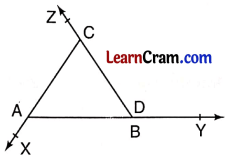∠CBY.
Angle CBY is the exterior angle of ΔABC on producing side AB to Y.

Question 2.
A, B, C, D are 4 points. Join them in pairs. How many triangles are formed?Number of triangles formed by joining the given points A, B, C and D in pair is ΔABC, ΔBCD, ΔCDA, ΔADB = 4 triangles.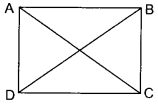Question 3.
Look at the figure and fill in the blanks.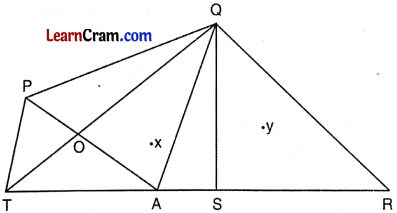(a) 2 triangles having point X in the exterior are ___________ and ___________.
ΔQAR and ΔPTA

(b) 2 triangles which have O as one vertex are ___________ and ___________.
ΔAOT and ΔPOQ

(c) 2 triangles which have PT as one side are ___________ and ___________.
ΔPTA and ΔPTQ

(d) 2 triangles having point Y in their interior are ___________ and ___________.
ΔQSR and ΔQAR

(e) 2 triangles which have A as one vertex are ___________ and ___________
ΔPAQ and ΔQASQuestion 3.
Fill in the blanks:
(a) The three ___________ of a triangle are non-collinear.
vertices

(b) Side opposite to the vertex C of ΔABC is ___________
AB

(c) Vertex opposite to the side XZ of ΔXYZ is ___________
Y

(d) The other two angles of a right angled triangle are ___________
acute

(e) Each angle of an equilateral triangle measures ___________
60°

### DAV Class 6 Maths Chapter 12 HOTS

Question 1.
In the given figure, find the sum of Answer:angles ∠A, ∠B, ∠C, ∠D, ∠E, ∠F.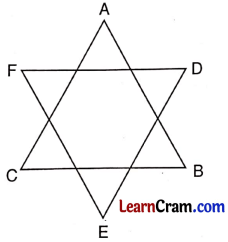In ΔABC,
∠A +∠B + ∠C = 180° …(i)
∠D + ∠E + ∠F = 180° …(ii)
On adding (i) and (ii), we get ∠A + ∠B + ∠C + ∠D + ∠E + ∠F
= 180° + 180°
= 360°Question 2.
Find the values of x and y.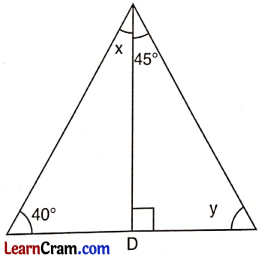Here, x + 40° + 90° – 180°
x + 130° = 180°
∴ x = 180° – 130°
= 50°
Again, y + 45° + 90° = 180°
⇒ y + 135° = 180°
∴ y = 180° – 135°
= 45°.

### DAV Class 6 Maths Chapter 12 Enrichment Questions

Question 1.
Find the sum of angles of the given polygon by dividing it into triangles.900°On dividing the polygon by joining its vertex it gives 5 triangles which makes a total of 5 × 180° = 900°.Question 2.
Compute the value of x, in the following figure.52°.
Linear pair of
120° = 180° – 120° = 60°
Now, 60° + x° = 112°
[∵ exterior angle = sum of opposite interior angles]
⇒ x° = 112° – 60° = 52°

Question 1.
Look at the figure and complete the statements: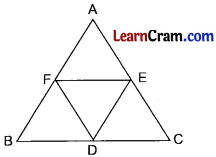(a) Number of all triangles are ___________.
5

(b) Sides opposite to vertex A are ___________ and ___________.
EF and BC

(c) Number of all the line segments is ___________.
12

(d) Triangles with common vertex B are ___________ and ___________.
ΔBFD and ΔBAC

(e) D is the vertex of triangle ___________, ___________ and ___________ .
ΔDEF, ΔBDF and ΔEDC

(f) A is the vertex of triangles ___________ and ___________.
ΔAEF and ΔABCQuestion 2.
Observe the given figure and answer the following.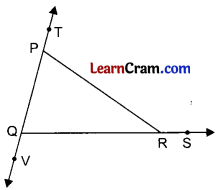(a) Name all the exterior angles
(b) Interior opposite angles of ∠PRS
(c) Adjacent interior angle of ∠RQV
(d) Interior opposite angles of ∠TPR
(e) Name the exterior angle formed by QV and QS.
(a) ∠PRS, ∠TPR and ∠VQR
(b) ∠QPR and ∠PQR
(c) ∠PQR
(d) ∠PQR and ∠PRQ
(e) ∠VQR

Question 3.
In which of the following conditions, the construction of the triangle is possible?
(a) Three angles of triangle are 40°, 60°, 80°.
(b) Three angles of triangle are 30°, 80°, 100°.
(c) The lengths of the 3 sides of a triangle are 6 cm, 3 cm, 11 cm.
(d) The three vertices of a triangle are collinear.
(e) The lengths of the three sides of a triangle are 5 cm, 12 cm and 13 cm.
(f) The three vertices of a triangle are non-collinear.
(a) Sum of the three angles is = 40° + 60° + 80° = 180°
Hence the triangle is possible

(b) Sum of the three angles is = 30° + 80° + 100 = 210° > 180°
Hence the triangle is not possible.

(c) Sum of the two smaller sides of the triangle = 6 + 3 = 9 cm
But 9 cm <11 cm
As the sum of two sides is less than the third side.
∴ triangle is not possible.

(d) Triangle is not possible to construct as the three vertices lie on the same straight line.

(e) Sum of two smaller sides = 5 + 12 = 17 cm > 13 cm.
Hence triangle is possible.

(f) Here the three vertices are non collinear
∴ Triangle is possible.

Question 4.
Fill in the blanks:
(a) Sum of all the angles of a triangle is ___________.
180°

(b) Sum of any two sides of a triangle is always ___________ than the third side.
greater

(c) Three vertices of a triangle must be ___________.
non-collinear

(d) The other two angles of a ___________ triangle are acute.
right angled

(e) The sides of a scalene triangle are ___________ in length.